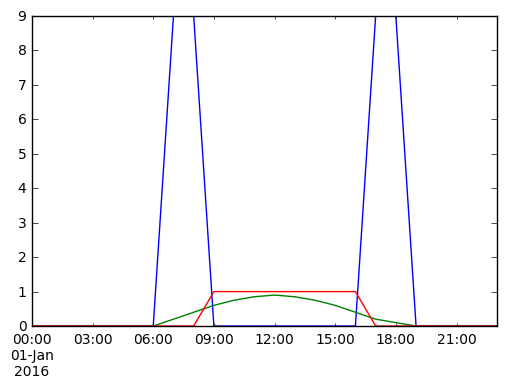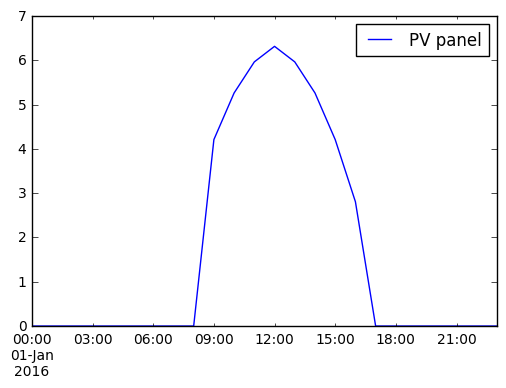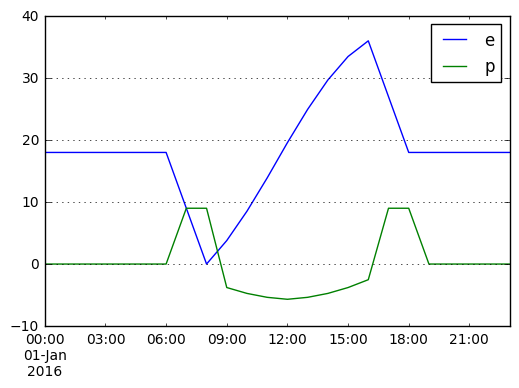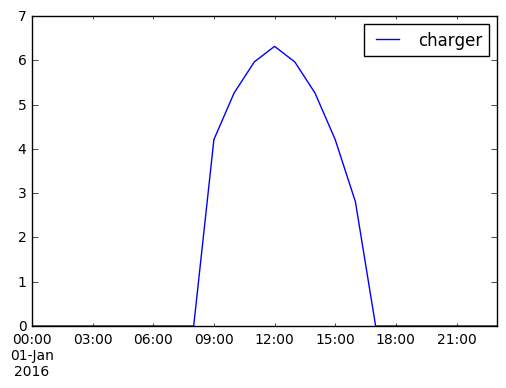# Battery Electric Vehicle Charging¶

In this example a battery electric vehicle (BEV) is driven 100 km in the morning and 100 km in the evening, to simulate commuting, and charged during the day by a solar panel at the driver's place of work. The size of the panel is computed by the optimisation.

The BEV has a battery of size 100 kWh and an electricity consumption of 0.18 kWh/km.

In :
import pypsa

import pandas as pd

%matplotlib inline

In :
# NB: this example will use units of kW and kWh, unlike the PyPSA defaults

# use 24 hour period for consideration
index = pd.date_range("2016-01-01 00:00","2016-01-01 23:00",freq="H")

# consumption pattern of BEV
bev_usage = pd.Series([0.]*7 + [9.]*2 + [0.]*8 + [9.]*2 + [0.]*5,index)

# solar PV panel generation per unit of capacity
pv_pu = pd.Series([0.]*7 + [0.2,0.4,0.6,0.75,0.85,0.9,0.85,0.75,0.6,0.4,0.2,0.1] + [0.]*5,index)

# availability of charging - i.e. only when parked at office
charger_p_max_pu = pd.Series(0.,index=index)
charger_p_max_pu["2016-01-01 09:00":"2016-01-01 16:00"] = 1.

In :
bev_usage.plot()
pv_pu.plot()
charger_p_max_pu.plot()

Out:
<matplotlib.axes._subplots.AxesSubplot at 0x7f7ef4e3be10>In :
network = pypsa.Network()

network.set_snapshots(index)

"place of work",
carrier="AC")

"battery",
carrier="Li-ion")

"PV panel",
bus="place of work",
p_nom_extendable=True,
p_max_pu=pv_pu,
capital_cost=1000.)

"driving",
bus="battery",
p_set=bev_usage)

"charger",
bus0="place of work",
bus1="battery",
p_nom=120,  #super-charger with 120 kW
p_max_pu=charger_p_max_pu,
efficiency=0.9)

"battery storage",
bus="battery",
e_cyclic=True,
e_nom=100.)

WARNING:pypsa.components:Attribute network.now is not in newly-defined snapshots. (network.now is only relevant if you call e.g. network.pf() without specifying snapshots.)

In :
network.lopf(network.snapshots)
print("Objective:",network.objective)

INFO:pypsa.pf:Slack bus for sub-network 0 is place of work
WARNING:pypsa.pf:No generators in sub-network 1, better hope power is already balanced
INFO:pypsa.pf:Slack bus for sub-network 1 is battery
INFO:pypsa.opf:Performed preliminary steps
INFO:pypsa.opf:Building pyomo model using angles formulation
INFO:pypsa.opf:Solving model using glpk
INFO:pypsa.opf:Optimization successful

('Objective:', 7017.54385964912)

In :
print("Pannel size [kW]:",network.generators.p_nom_opt["PV panel"])

('Pannel size [kW]:', 7.0175438596491198)

In :
network.generators_t.p.plot()

Out:
<matplotlib.axes._subplots.AxesSubplot at 0x7f7ef28f9310>In :
pd.DataFrame({attr: network.stores_t[attr]["battery storage"] for attr in ["p","e"]}).plot(grid=True)

Out:
<matplotlib.axes._subplots.AxesSubplot at 0x7f7ef2843b50>In :
print("Losses [kWh/d]:",network.generators_t.p.loc[:,"PV panel"].sum() - network.loads_t.p.loc[:,"driving"].sum())

('Losses [kWh/d]:', 3.9999999999999716)

In :
network.links_t.p0.plot()

Out:
<matplotlib.axes._subplots.AxesSubplot at 0x7f7ef277af10>